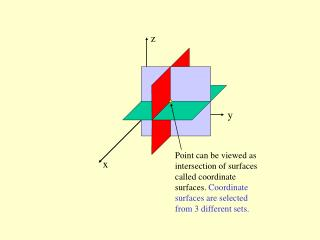DownloadDownload PresentationPoint can be viewed as intersection of surfaces called coordinate surfaces. Coordinate surfaces are selected from 3 dif

# Point can be viewed as intersection of surfaces called coordinate surfaces. Coordinate surfaces are selected from 3 dif

Download Presentation## Point can be viewed as intersection of surfaces called coordinate surfaces. Coordinate surfaces are selected from 3 dif

- - - - - - - - - - - - - - - - - - - - - - - - - - - E N D - - - - - - - - - - - - - - - - - - - - - - - - - - -
##### Presentation Transcript

1. z y Point can be viewed as intersection of surfaces called coordinate surfaces. Coordinate surfaces are selected from 3 different sets. x

2. z y x Example of first set of coordinate surfaces

3. z y x Example of second set of coordinate surfaces

4. z y x Example of third set of coordinate surfaces

5. z y x Any surface in one set should be orthogonal to any surface in any other set

6. Every coordinate system requires a special point called the origin in order to define position of the coordinate surfaces

7. z y x Frequently one needs to describe a vector field (vector valued function of position). In this case it is convenient to introduce coordinate vectors associated with every point in space.

8. z y x First coordinate vector

9. z y x Second coordinate vector

10. z y x Third coordinate vector

11. z y x Coordinate system where all coordinate surfaces are planar is called Cartesian. Note that in this system direction of coordinate vectors is the same at every point in space

12. In a cylindrical coordinate system, a cylindrical surface and two planar surfaces all orthogonal to each other define location of a point in space. The coordinate vectors are and . Note, in this system the direction of coordinate vectors changes from point to point

13. In a spherical coordinate system the surfaces of spheres, cones and planes are coordinate surfaces. The corresponding coordinate vectors also change their direction from point to point

14. z y x Transformations between cylindrical and Cartesian From Cartesian to cylindrical From cylindrical to Cartesian

15. Transformations between spherical and Cartesian z From Cartesian to spherical y x From spherical to Cartesian

16. z y x Since the choice of vectors normal to a surface is not unique, the choice of coordinate vectors is also not unique. What is most important, however, is not the absolute direction of the coordinate vectors, but their directions relative to each other.

17. z y x By convention, we will choose relative directions of the coordinate vectors to satisfy the “right hand” rule. First choose one coordinate vector, say vector x. Point your index finger along this vector. Now, stick our your middle finger and your thumb in directions perpendicular to your index finger. These should be the directions of the second and third coordinate vectors, respectively.